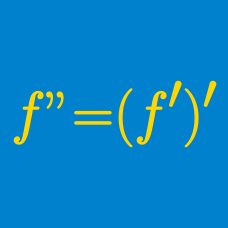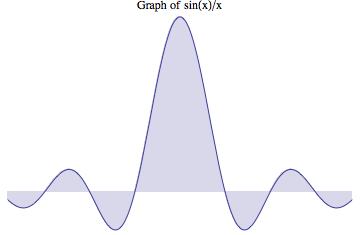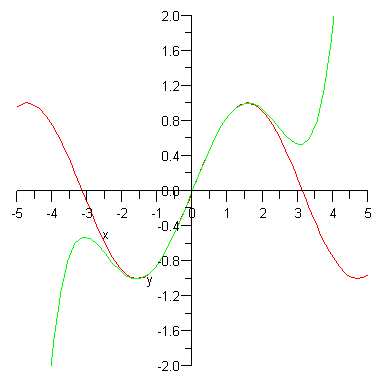Calculus

# Higher-order Derivatives: Level 4 Challenges

What is the third derivative of $\large x^{x^x}$ at $x=1?$In mathematics, physics, and engineering, the cardinal sine function or sinc function, denoted by $\def\sinc{\text{sinc }} \sinc x$, is defined by $\def\sinc{\text{sinc }} \sinc x=\frac{\sin x}{x}.$ The term "sinc" is a contraction of the function's full Latin name, the sinus cardinalis (cardinal sine). It was introduced by Phillip M. Woodward in his 1952 paper "Information Theory and Inverse Probability in Telecommunication" in which he said the function "occurs so often in Fourier analysis and its applications that it does seem to merit some notation of its own" and his 1953 book "Probability and Information Theory, with Applications to Radar".

Compute $m+n$, where $m$ and $n$ are relatively prime, where

$\def\sinc{\text{sinc }} \left.\frac{d^{100}}{dx^{100}}(\sinc x)\right|_{x=0}=\frac{m}{n}.$$f'(x) = f'''(x)$

For some infinitely differentiable function $f(x)$, it satisfies the above equation. Given the initial conditions

$f(1) = 6, f'(1) = 5, f''(1) = -3$

What is the value of $f( \ln(42) )$?

Details and Assumptions

$f'(k), f''(k), f'''(k)$ denote the first, second, and third derivative of $f(x)$ at $x=k$ respectively.

###### Image Credit: Wikimedia Taylor Series$\large \frac{d^n}{dx^n} \frac{\ln(x)}{x} = \frac{a_n\ln(x)-b_n}{x^{n+1}}$

Let $f^{(n)}(x)$ be defined as the $n$-th derivative of $\frac{\ln(x)}{x}$.

If $f^{(n)}(x)$ can be written in the form shown above, then the solution to $f^{(n)}(x)=0$ can be written in the form $x=e^{\frac{p_n}{q_n}}$ where $p_n$ and $q_n$ are coprime positive integers.

What is $p_{10}+q_{10}$?

$\LARGE x^{x^{x^{x^{x^{x^x}}}}}$

Find the third derivative of the function above at $x=1$.

×